# bfd

MATLAB code for Bayesian interpretation of Fisher's discriminant

View the Project on GitHub lawrennd/bfd

# Bayesian Fisher's discriminant

This page describes how to use the so-called Bayesian Fisher Discriminant (BFD) software.

Please note that in the subsequent discussion we will be using these references:

1. T. Peña-Centeno and N. D. Lawrence. (2006) "Optimising kernel parameters and regularisation coefficients for non-linear discriminant analysis" in Journal of Machine Learning Research, accepted for publication.

2. T. Peña-Centeno and N. D. Lawrence. (2004) "Optimising kernel parameters and regularisation coefficients for non-linear discriminant analysis" Technical Report no CS-04-13, University of Sheffield, Department of Computer Science.

3. Kevin J. Lang and Michael J. Witbrock (1988) "Learning to tell two spirals apart" in Proceedings of the 1988 Connectionist Summer School. Morgan Kauffman

4. Spiral data, available here.

5. Gunnar Rätsch's data.

### Release Information

Current release is 0.12

Release 0.12 was used for the experiments presented in . The code was written with flexibility in mind so probably there is room to make improvements in terms of efficiency of computation.

Release 0.11 corresponds to the results presented in .

The BFD software requires some functions, written by Neil D. Lawrence, that have been put together in form of the NDLUTIL toolbox. You may access this toolbox with the username and password you are given when you register for the BFD toolbox. There are many versions of this toolbox but we recommend only a few of them in order to obatin the results of .

Toolbox Version
NDLUTIL 0.12 (or below)
Netlab 3.3 (It should work with v3.2 as well)
ls-SVMlab 1.5

To obtain the classification results for benchmark data it will be necessary to download Gunnar Rätsch's data, available at . You will also need the function normal, written by Ian T. Nabney. Note: this function does not belong to the Netlab toolbox, but is rather a worked example.

## Examples

### Synthetic datasets

The experiments with synthetic data, presented in , can be recreated by using the function `bfdClassifyToyData`. This function can be applied on the four synthetic datasets included in the toolbox, henceforth referred as: spiral, overlap, bumpy and relevance. `bfdClassifyData` also allows the use several type of GP priors, among them an RBF and an ARD-composed one. The spiral problem was first proposed by  and the dataset included in this distribution is based on the file found at .

Given the inputs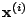and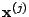we have implemented the RBF prior in the following way,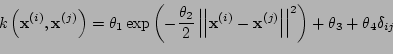,

where the parameter vector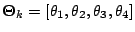.

The ARD-composed kernel is defined as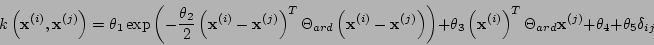,

with a vector of parameters given by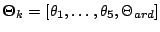. This kernel is composed of four parts: RBF-ARD, LINEAR-ARD, BIAS and NOISE parts, thus giving its name of 'composed'.

#### RBF experiments

Just run the following commands from Matlab's command prompt

```>> dataset = 'overlap';
>> kernelType = {'rbf', 'bias', 'white'};
>> model = bfdClassifyToyData(dataset, kernelType);       ````

For each dataset (`spiral`, `overlap`, `bumpy` and `relevance`) these commands should produce the plots shown below. The function `bfdClassifyToyData` also returns a structure model containing all the information about the discriminant.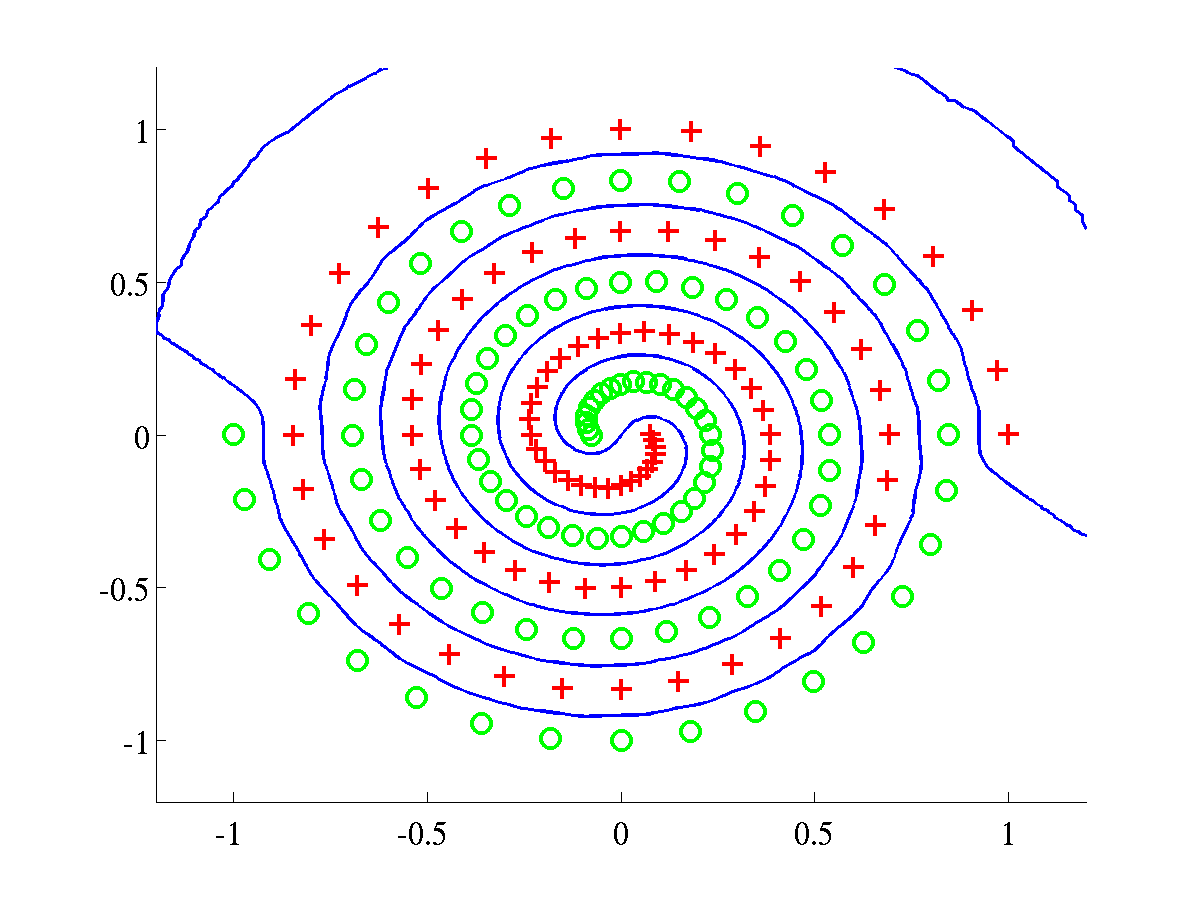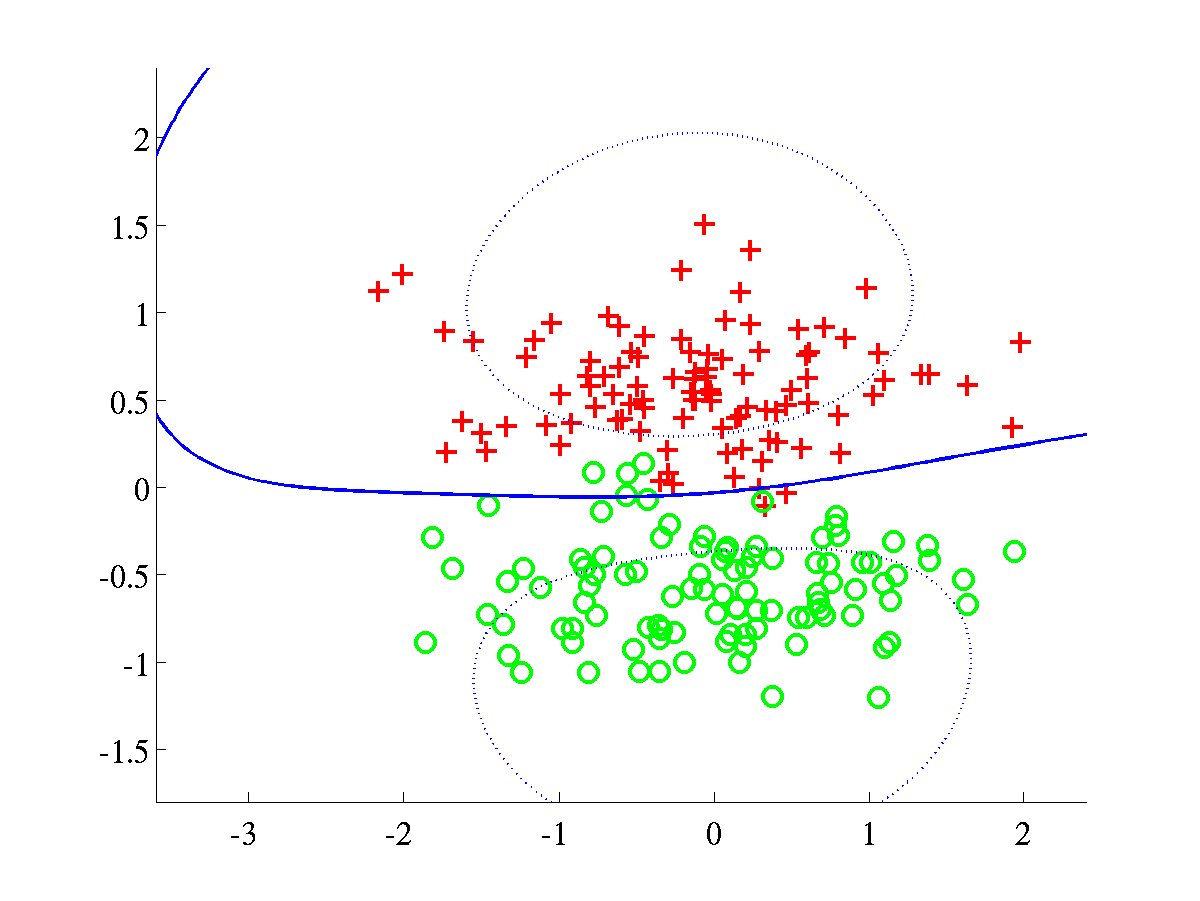Classification of synthetic datasets using an RBF kernel. Two classes are shown as pluses and circles. The separating line, in blue, was obtained by projecting test data over a grid. The dotted line indicates points at 1/4 of the distance, as measured in projected space, from the decision boundary to the class mean. The blue line was obtained after training a BFD model by maximising the marginal log-likelihood, L.   Left: Spiral data, with bound after training L=658.6107.   Right: Overlap data with final bound L=638.5258. In both problems the parameters were initialised to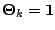.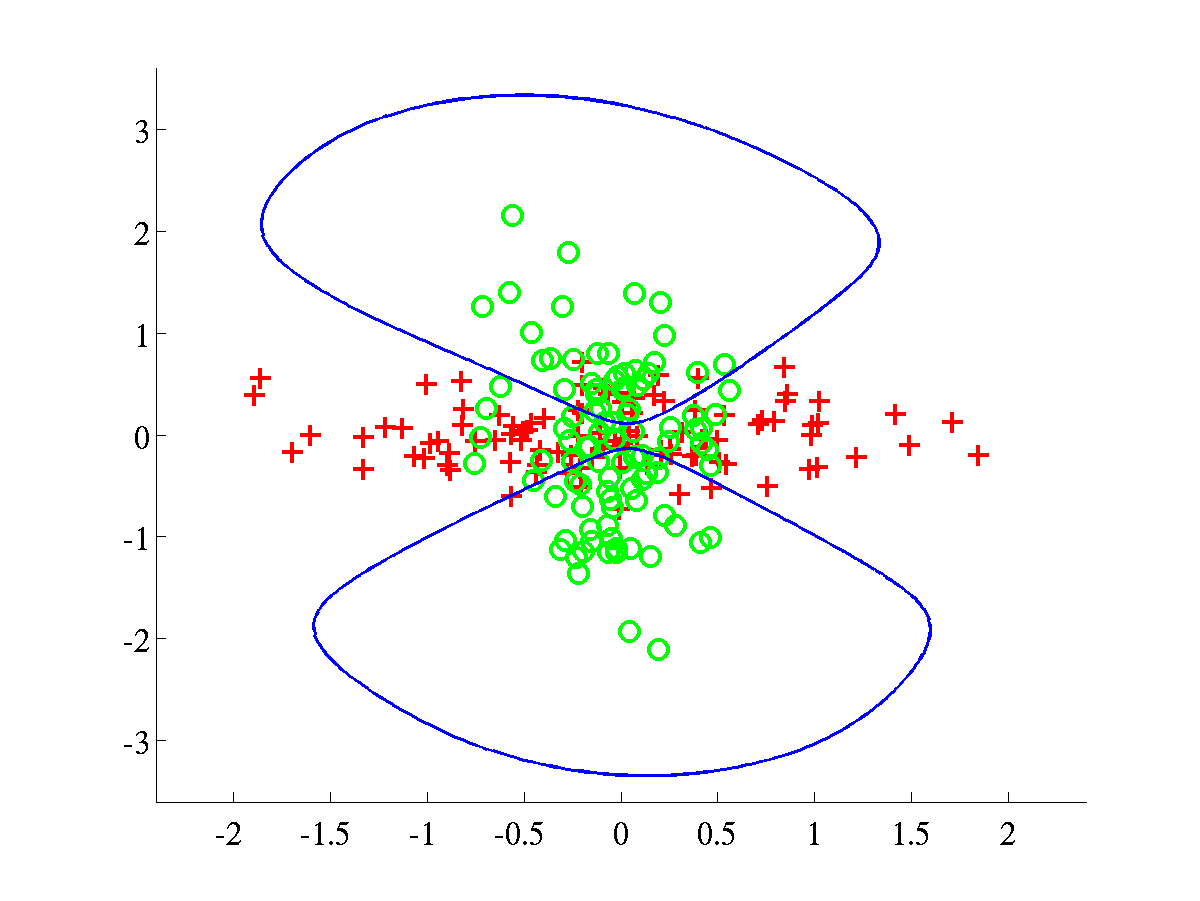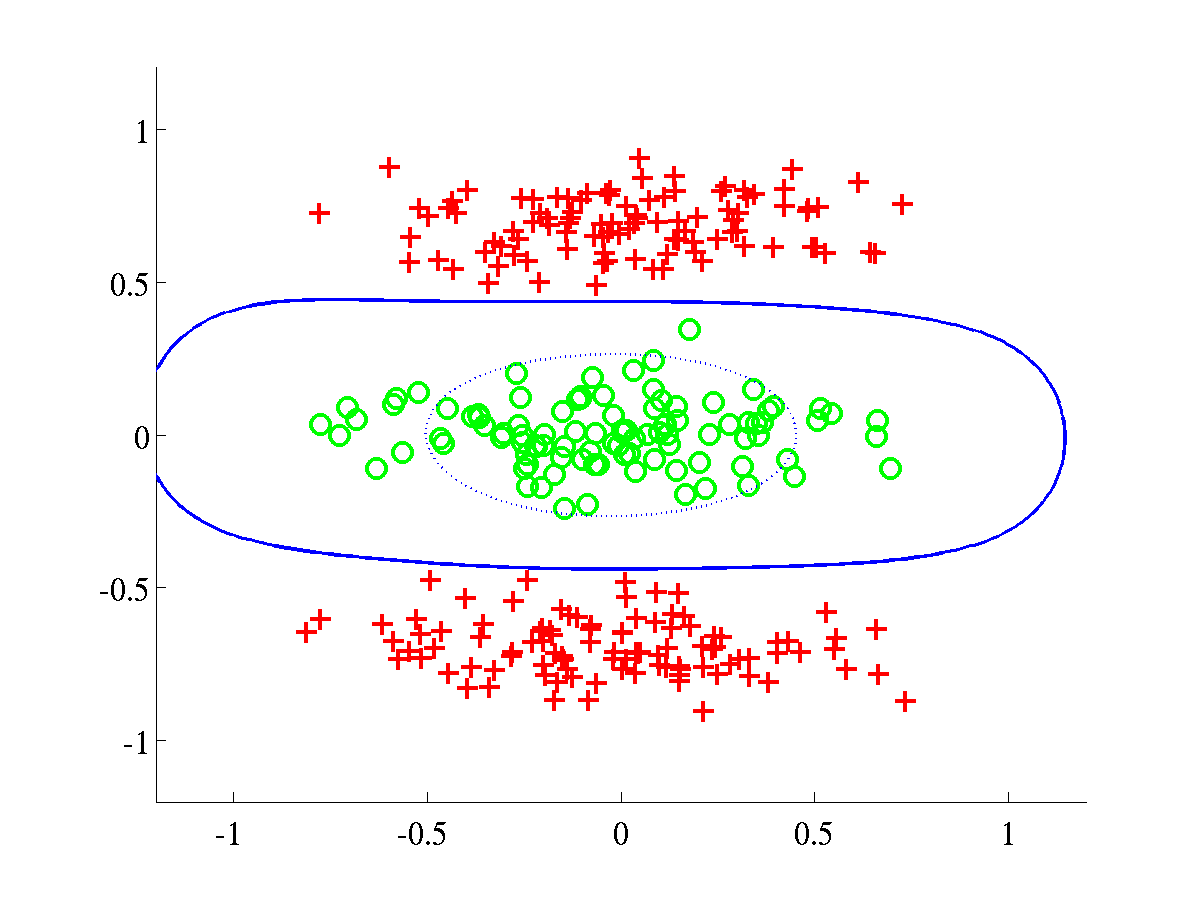Left: Bumpy data with final bound L=609.0547.   Right: Relevance data, with bound L=895.7308. For both problems the initial parameters were set to.

#### ARD experiments

In order to run experiments with the ARD-composed kernel, it is necessary to use a different value for the variable `kernelType`, as it is shown below.

```>> dataset = 'spiral';
>> kernelType = {'rbfard', 'linard', 'bias', 'white'};
>> model = bfdClassifyToyData(dataset, kernelType);       ````

The script should produce the plots shown below.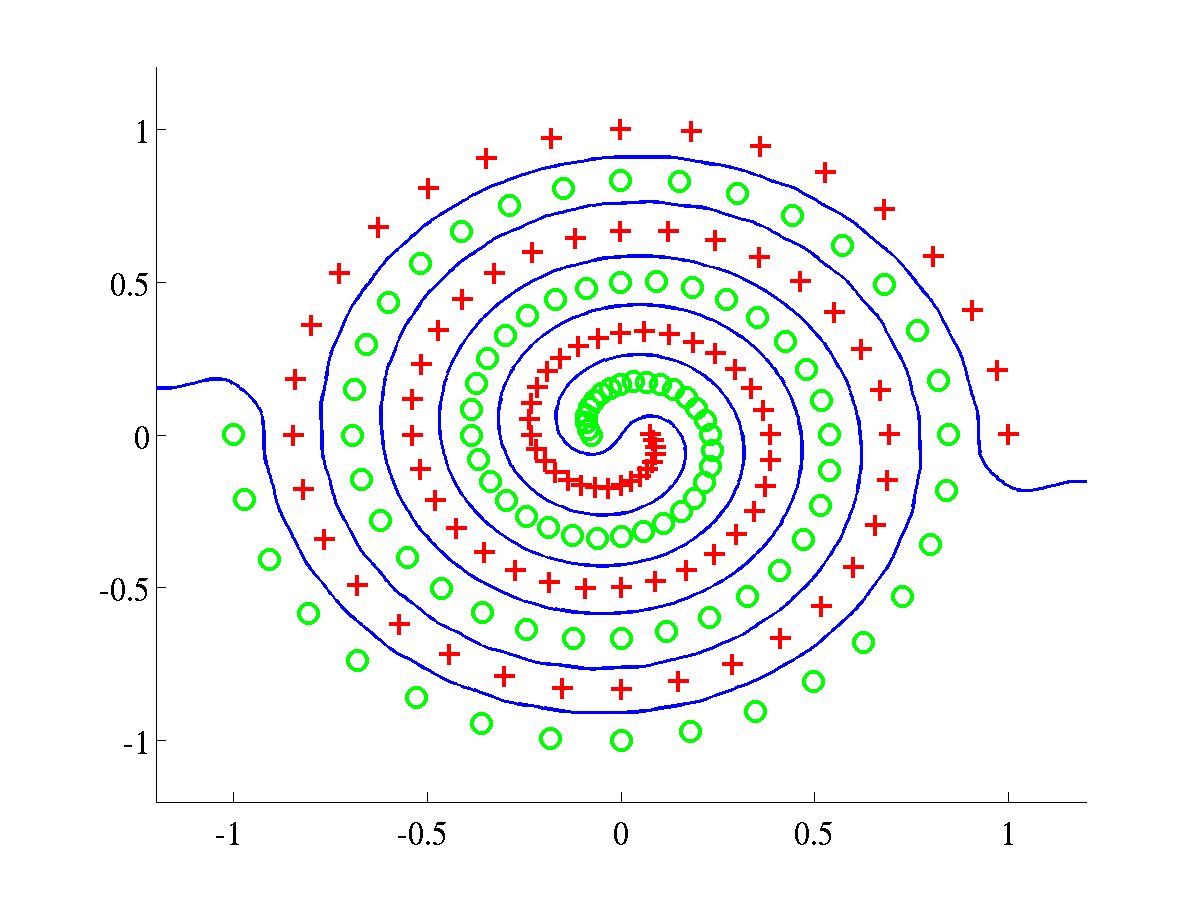Left: Spiral data, with final L=674.2042. In this experiment we initialised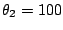and the rest of parametersRight: Overlap data, with final L=650.0908. This experiment used as initial parameters.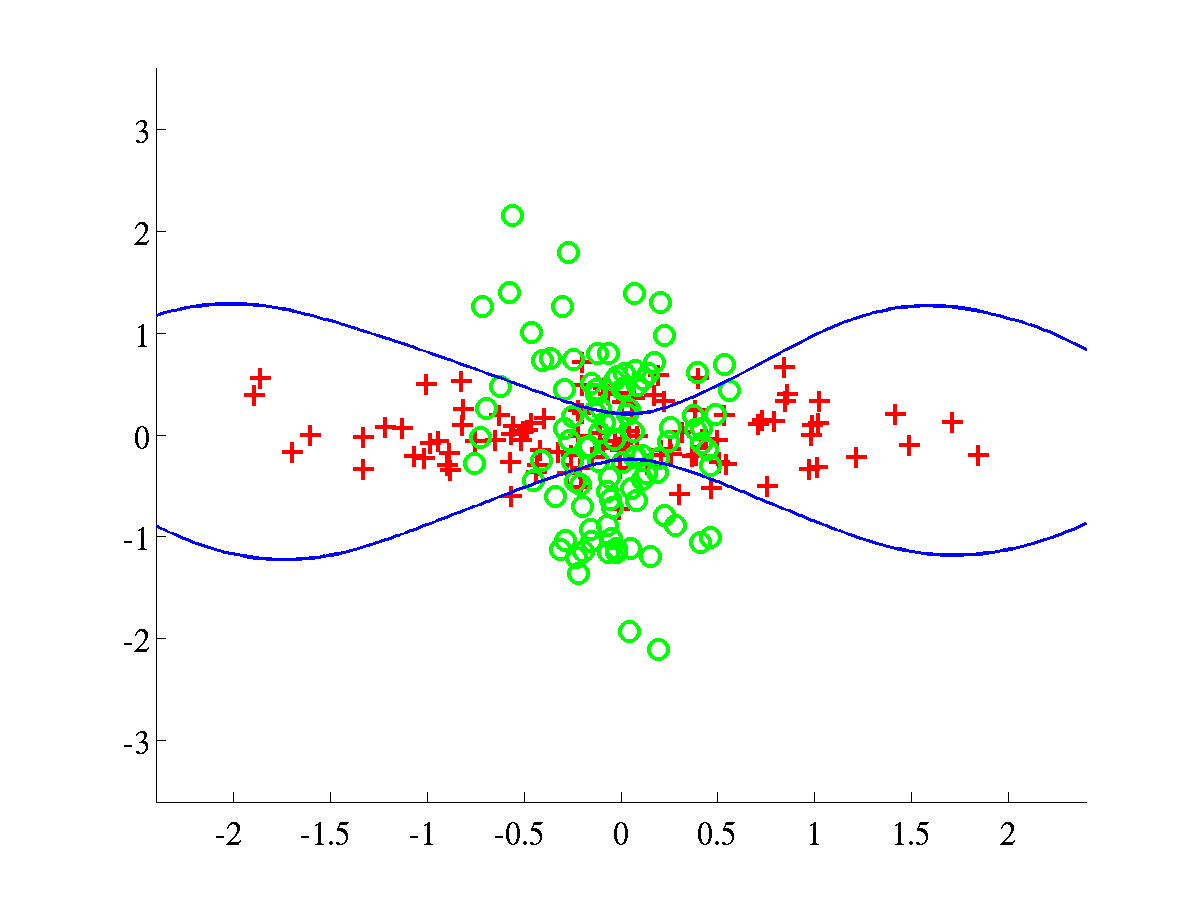Left: Bumpy data, with final L=636.8442.   Right: Relevance data, with final L=998.9992. In both problems we used an initial.

#### Generating ROC curves and computing AUC's

There are several demos included in this distribution and two of them were specifically written to obtain some of the results presented in Table 1 of reference . More specifically, these demos generate ROC curves for either banana or breast-cancer data and then obtain statistics related to the AUC's (area under ROC curves).

The first demo can be run from the command-line as shown below

``````>> demAUCbanana
``````

After execution, a file

`banana_rbfbiaswhite_test_AUC_info.txt`

will be created inside the directory `<bfd-path>/bananaROC/`. This file contains several statistics related to the ROC curves of banana and should look like this

``````Dataset: banana
Kernel used: rbfbiaswhite
These are some statistics of the ROC curves
###########################################
mean = 0.950725
median = 0.952039
max = 0.960705
min = 0.929625
std = 0.006099
``````

Further information in the directory `<bfd-path>/bananaROC/` will be stored, for example a copy of the ROC curve for every test instance of banana. The second demo does the analogue process for breast-cancer. Hence it is required to run

`>> demAUCbreastCancer  `

in order to obtain a result of this kind

``````Dataset: breast-cancer
Kernel used: rbfbiaswhite
These are some statistics of the ROC curves
###########################################
mean = 0.734383
median = 0.736931
max = 0.909649
min = 0.615699
std = 0.052524.
``````

#### Note on ROC curves

During execution of either of the scripts (`demAUCbanana` or `demAUCbreastCancer`) the ROC curve of each test instance of the datasets will be shown on the screen for a couple of seconds. This should look as shown below.Some ROC curves of the test instances of banana. Left: First instance. Right: Second instance.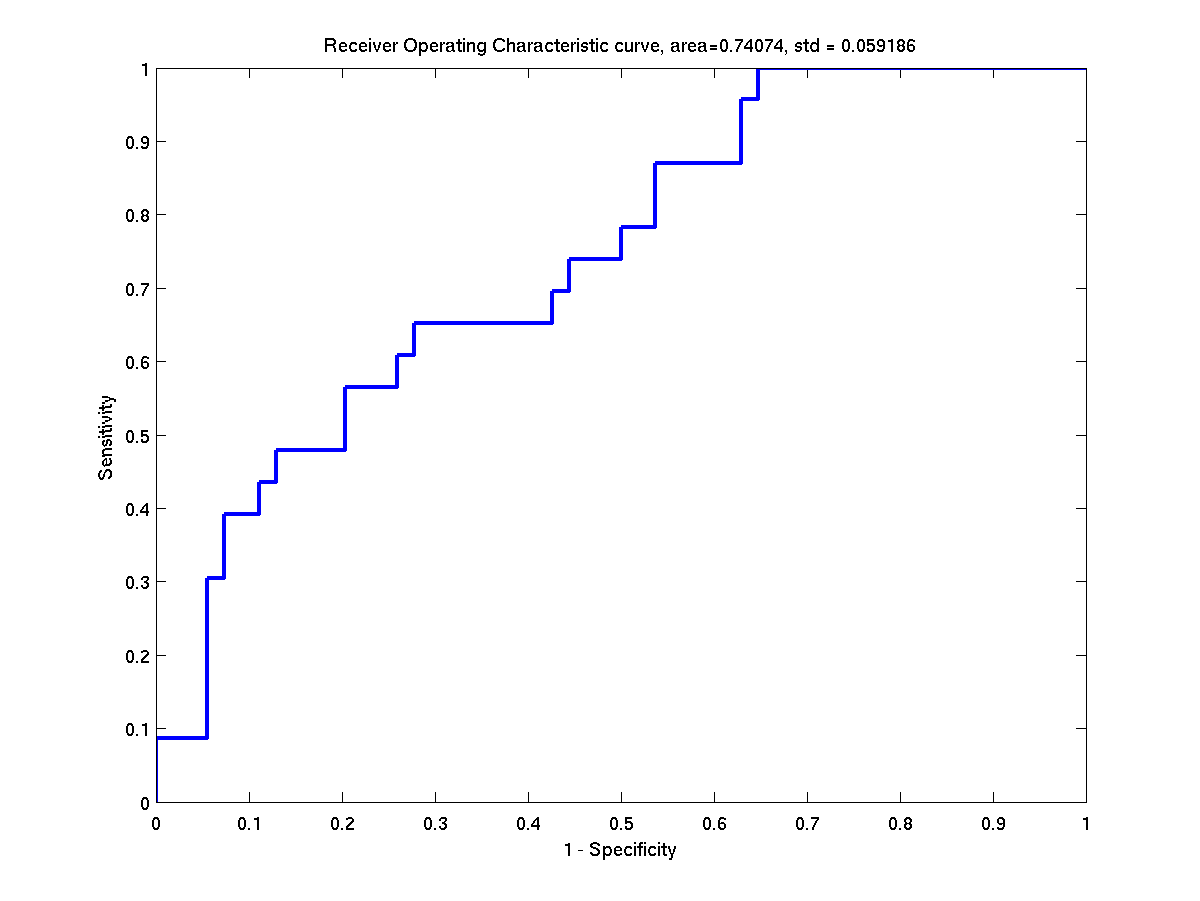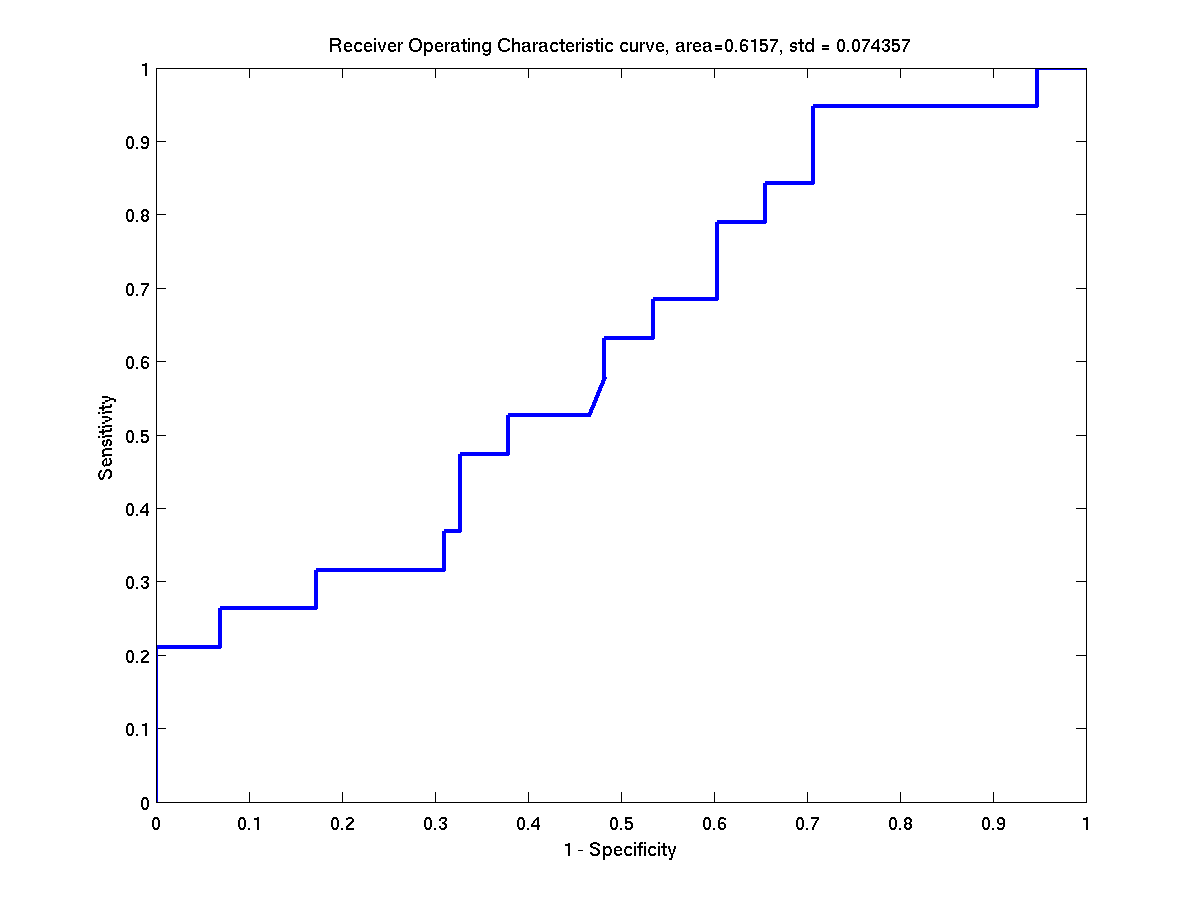Some ROC curves of the test instances of breast-cancer. Left: First instance. Right: Second instance.

An extra script obtains ROC curves (and additional statistics) for the heart dataset; this time according to the results of Table 2 of reference . Again, it is only a matter of running a script.

``````>> demAUCheart
``````

The result file should look as follows.

``````Dataset: heart
Kernel used: rbfardlinardbiaswhite
These are some statistics of the ROC curves.
###########################################
mean = 0.909429
median = 0.908183
max = 0.960591
min = 0.845779
std = 0.027045
``````

#### Generating histograms of projected data

The histograms presented in Figure 10 of reference  can be created by running two scripts. The first script

``````>> demHistTwonorm
``````

will display and save on file the histograms of twonorm projected data. All files related the histograms of twonorm will be stored in a directory `<bfd-path>/twonorm_avgHistogram`. Among other things, a couple of `.eps` files will contain the average histograms of training and test data. Such histograms should be like the ones below.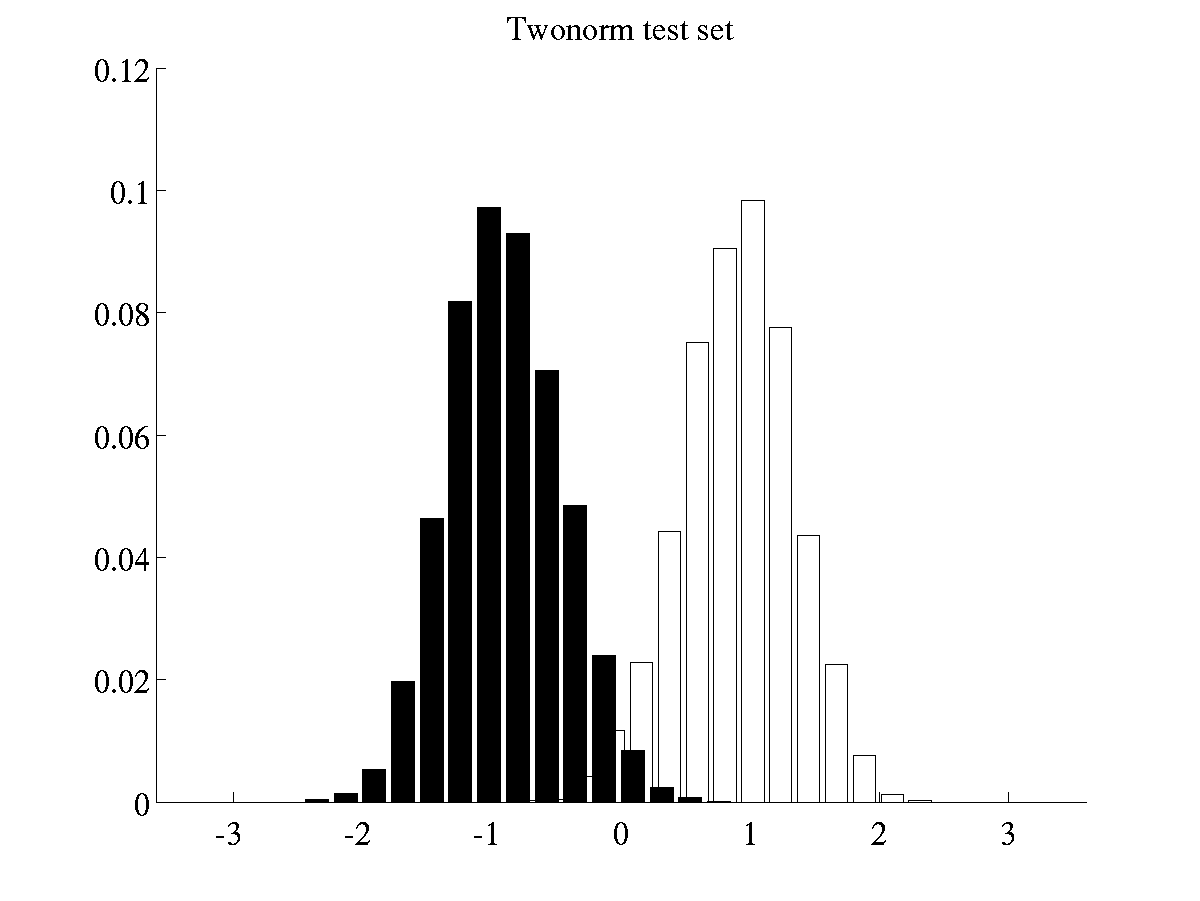Left: Histogram of projected data for training instances of twonorm Right: Histogram of projected data for test instances of twonorm. The batch of histograms of waveform are obtained by running the script

``````>> demHistWaveform
``````

which generates plots like the following ones.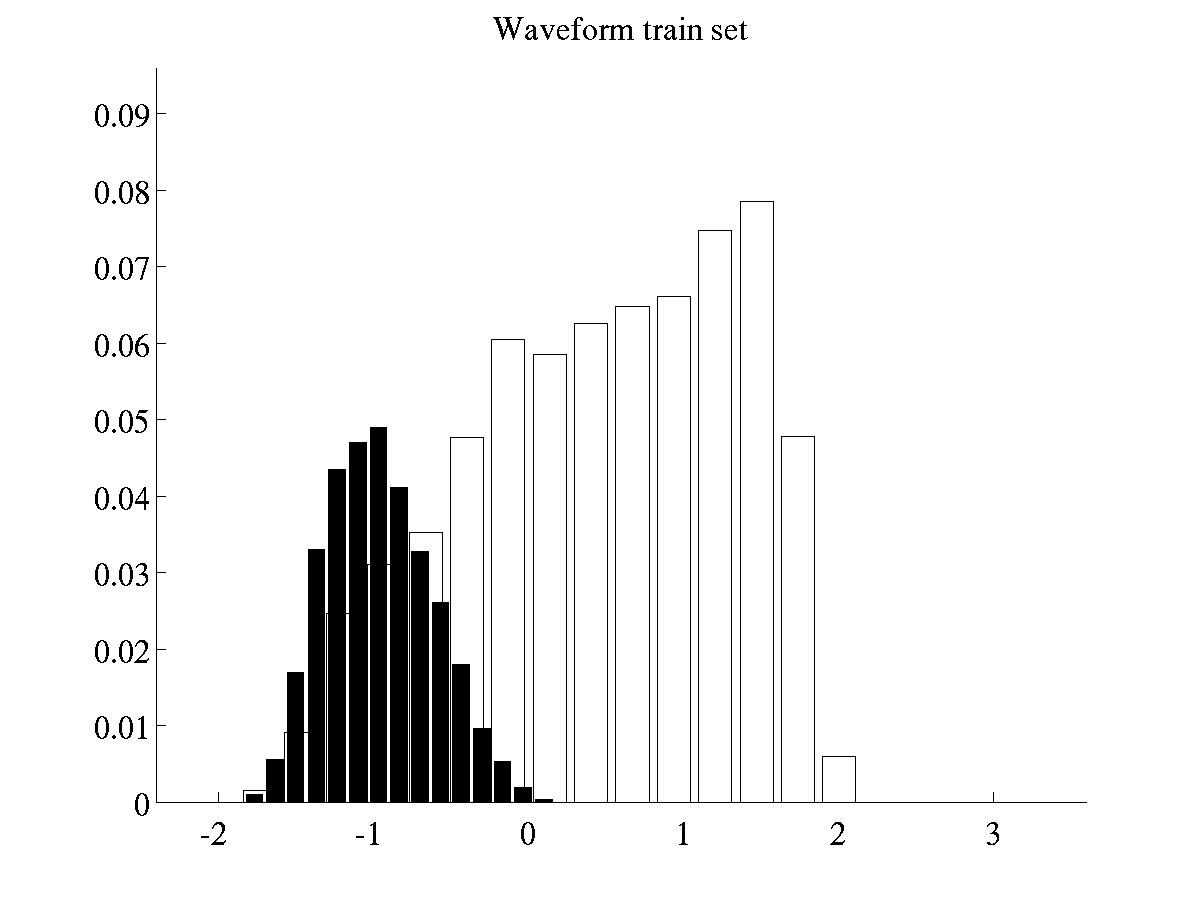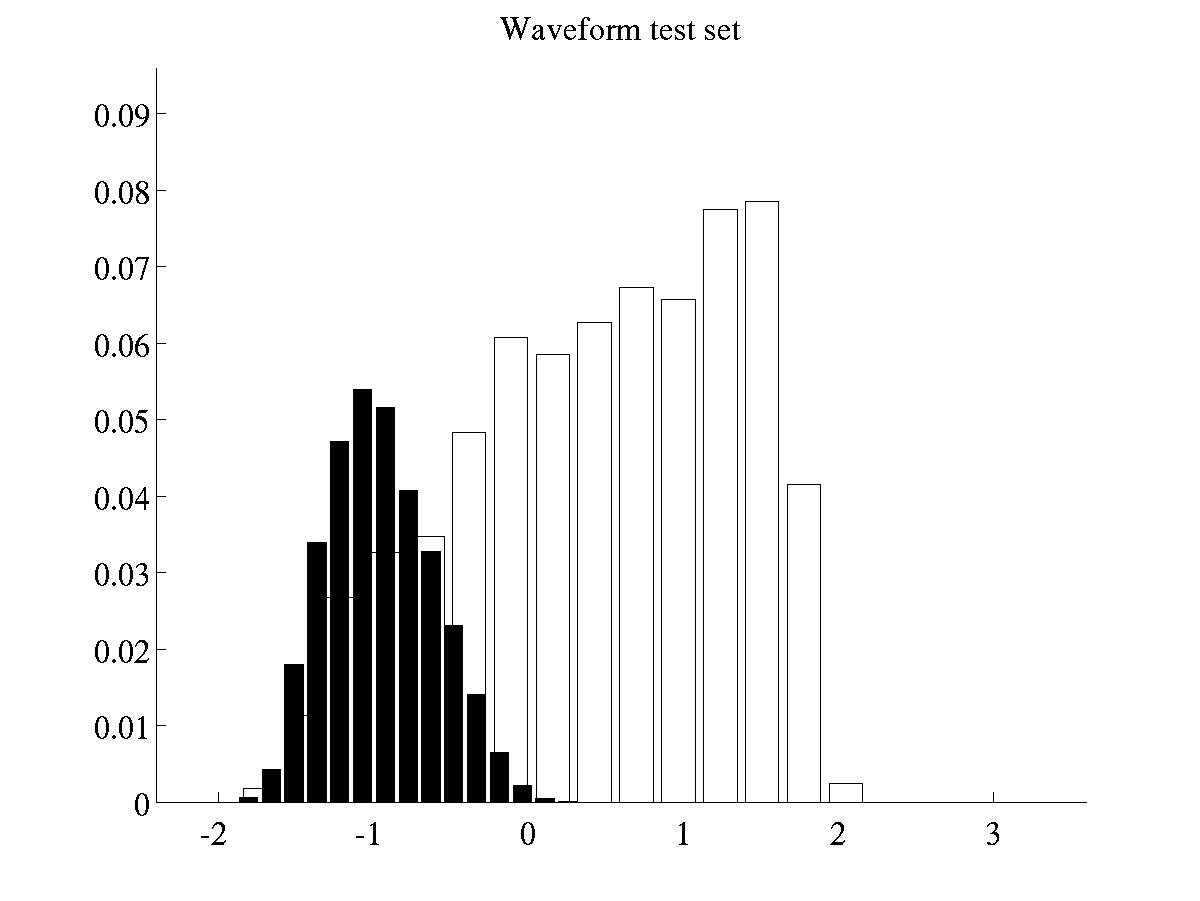Left: Histogram of projected data for training instances of waveform Right: Histogram of projected data for test instances of waveform

#### Other experiments

Further experiments can be carried out by modifying the scripts previously mentioned of by using the functions `bfdClassifyData` and `bfdClassifyToyData`. Please note that in order to recreate the results of , it will also be necessary to read the file experimentResults.txt included in this distribution.

Tonatiuh Pena Centeno 2006-02-11